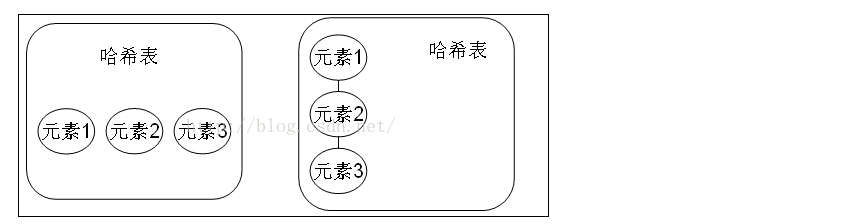# 【Java Set】1.Set集合的详解

Set注重独一无二的性质，该体系集合可以知道某物是否已经存在于集合中，不会存储重复的元素，用于存储无序（存入和取出的顺序不一定相同）元素，值不能重复

/**
* Collection
*      \--List
*          有序（存储顺序和取出顺序一致），可重复
*      \--Set
*          无序（存储顺序和取出顺序不一致），唯一
* HashSet:它不保证set的迭代顺序；特别是它不保证该顺序恒久不变
* 注意：虽然set集合的元素无序，但是，作为集合来说，它肯定有它自己的存储顺序，
* 而你的顺序恰巧和它的存储顺序一致，这代表不了有序，你可以多存储一些数据就能看到效果
**/

    public static void main(String[] args) {
//创建集合对象
Set<String> set=new HashSet<String>();
//创建并添加元素
set.add("hello");
set.add("world");
set.add("java");
set.add("java");
set.add("apple");
//增强for循环 [world,java,hello,apple]
for (String s:set){
System.out.println(s);
}
}

HashSet是一个没有重复元素的集合，它其实是由HashMap实现的，HashMap保存的是建值对，然而我们只能向HashSet中添加Key，原因在于HashSet的Value其实都是同一个对象，这是HashSet添加元素的方法，可以看到辅助实现HashSet的map中的value其实都是Object类的同一个对象。

    public static void main(String[] args) {
//创建集合对象
HashSet<String> hashSet=new HashSet<String>();
//创建并添加元素
hashSet.add("hello");
hashSet.add("world");
hashSet.add("java");
hashSet.add("java");
//遍历集合 [java,hello,world]
for(String s:hashSet){
System.out.println(s);
}
}

interface Collection{
...
}

interface Set extends Collection{
...
}

class HashSet implements Set{

}

class HashSet implements Set{
private static final Object PRESENT = new Object();
private transient HashMap<E,Object> map;
public HashSet() {
map = new HashMap<>();
}

public V put(K key, V value) {
return putVal(hash(key), key, value, false, true);
}

final V putVal(int hash, K key, V value, boolean onlyIfAbsent,
boolean evict) {
Node<K,V>[] tab; Node<K,V> p; int n, i;
if ((tab = table) == null || (n = tab.length) == 0)
n = (tab = resize()).length;
if ((p = tab[i = (n - 1) & hash]) == null)
tab[i] = newNode(hash, key, value, null);
else {
Node<K,V> e; K k;
if (p.hash == hash &&
((k = p.key) == key || (key != null && key.equals(k))))
e = p;
else if (p instanceof TreeNode)
e = ((TreeNode<K,V>)p).putTreeVal(this, tab, hash, key, value);
else {
for (int binCount = 0; ; ++binCount) {
if ((e = p.next) == null) {
p.next = newNode(hash, key, value, null);
if (binCount >= TREEIFY_THRESHOLD - 1) // -1 for 1st
treeifyBin(tab, hash);
break;
}
if (e.hash == hash &&
((k = e.key) == key || (key != null && key.equals(k))))
break;
p = e;
}
}
if (e != null) { // existing mapping for key
V oldValue = e.value;
if (!onlyIfAbsent || oldValue == null)
e.value = value;
afterNodeAccess(e);
return oldValue;
}
}
++modCount;
if (++size > threshold)
resize();
afterNodeInsertion(evict);
return null;
}
public boolean add(E e) {
return map.put(e, PRESENT)==null;
}
}

HashSet：哈希表里面存放的是哈希值，HashSet存储元素的顺序并不是按照存入时的顺序（和List显然不同） 是按照哈希值来存的所以取数据也是按照哈希值取得。HashSet不存入重复元素的规则.使用hashcode和equals，由于Set集合是不能存入重复元素的集合。那么HashSet也是具备这一特性的。HashSet如何检查重复？HashSet会通过元素的hashcode（）和equals（）方法进行判断元素师否重复，当你试图把对象加入HashSet时，HashSet会使用对象的hashCode（）方法来判断对象加入的位置。同时也会与其他已经加入的对象的hashCode进行比较，如果没有相等的hashCode，HashSet就会假设对象没有重复出现，简单一句话，如果对象的hashCode值是不同的，那么HashSet会认为对象是不可能相等的，因此我们自定义类的时候需要重写hashCode，来确保对象具有相同的hashCode值。

HashTableHashSet：通过hashCode值来确定元素在内存中的位置。一个hashCode位置上可以存放多个元素。

HashSet 和ArrayList集合都有判断元素是否相同的方法，

boolean contains(Object o)

HashSet使用hashCode和equals方法，ArrayList使用了equals方法